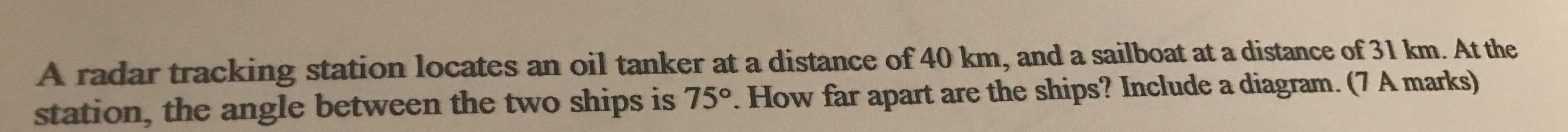### Still have math questions?A radar tracking station locates an oil tanker at a distance of $$40 km$$ , and a sailboat at a distance of $$31 km$$ . At the station, the angle between the two ships is $$75 ^ { \circ }$$ . How far apart are the ships?